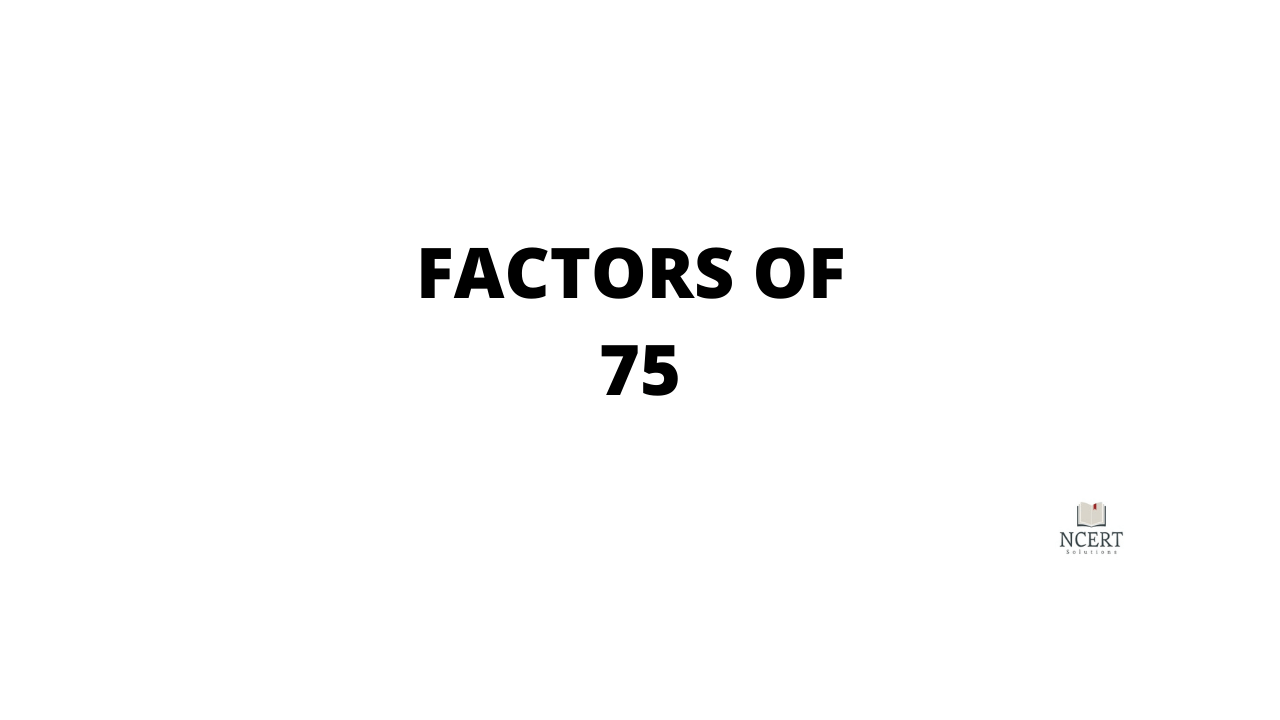## Factors of 75

The factors of NS 75 are the numbers which when two numbers are multiplied together give the result of 75. Pair factors of 75 are whole numbers that can be either positive or negative but not fractions or decimal numbers. To find the factors of a number, 75, we can use the two methods – …